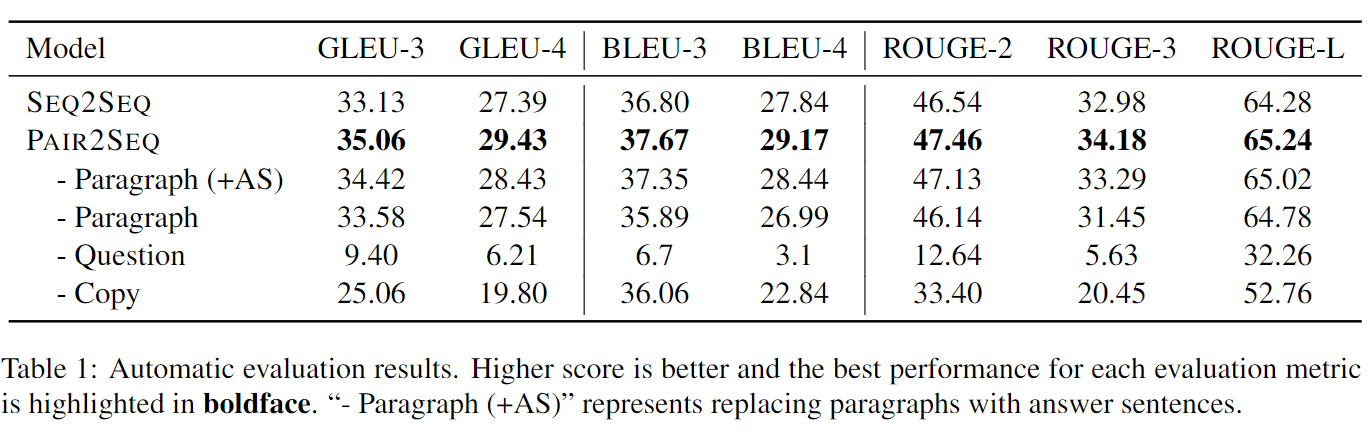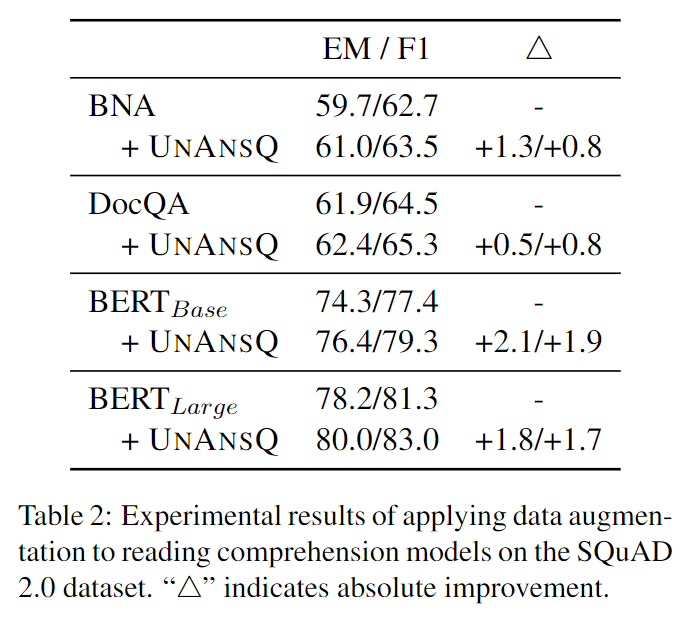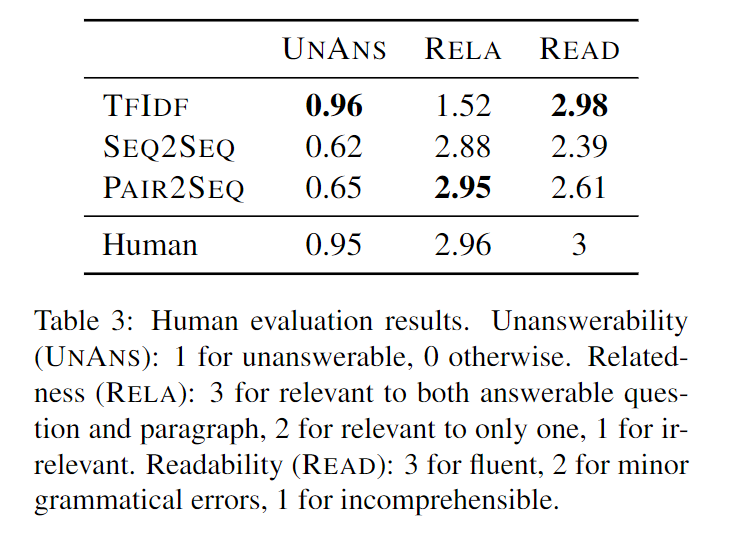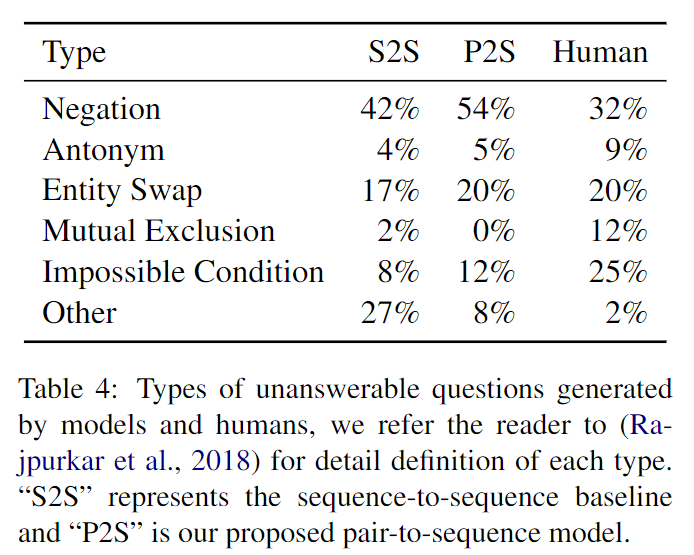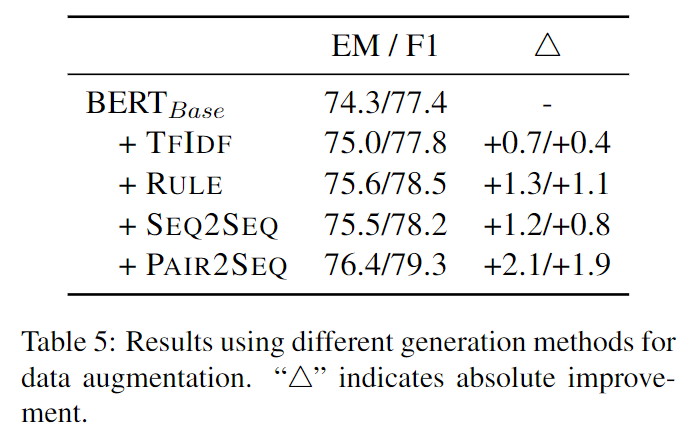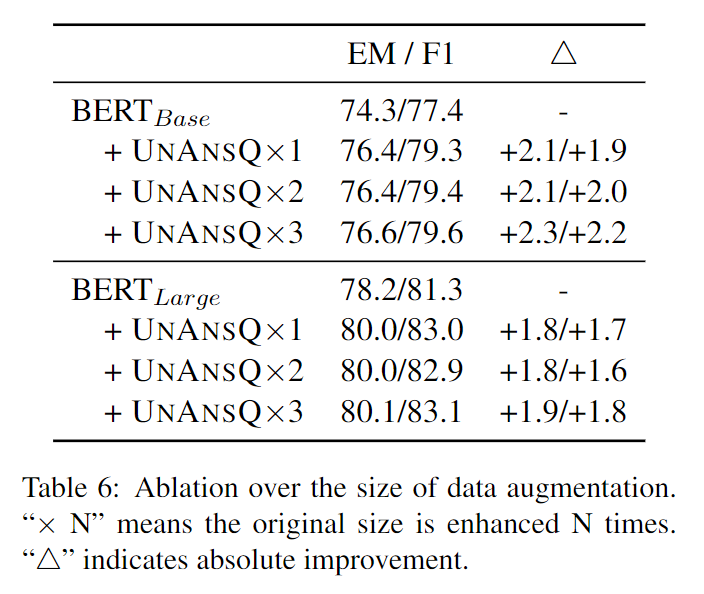# 机器阅读理解中数据增强笔记

2019/10/29

## 数据观察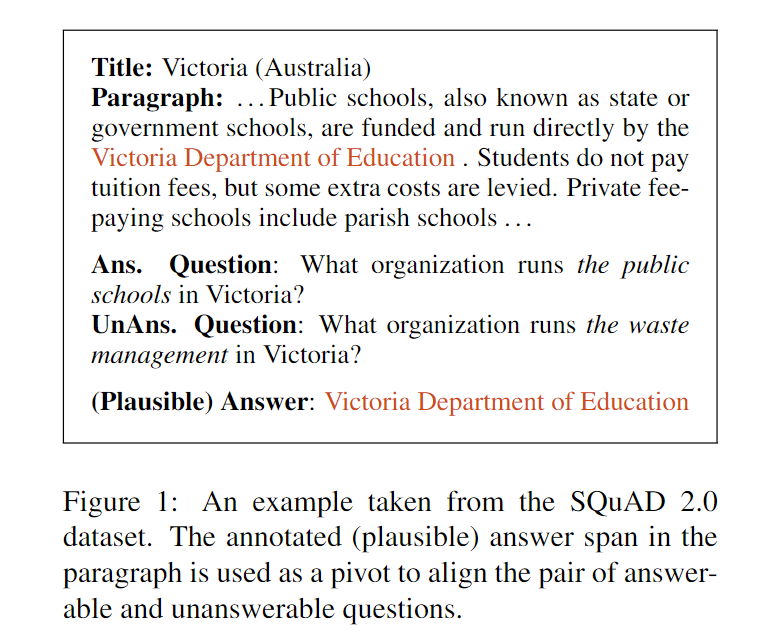## 问题描述

• 问题$\tilde{q}$在段落$p$中找不到答案
• 问题$\tilde{q}$需要与可回答的问题$q$和段落$p$都相关，这可避免产生不相关的问题
• 问题$\tilde{q}$需问一些和答案$a$相关的问题。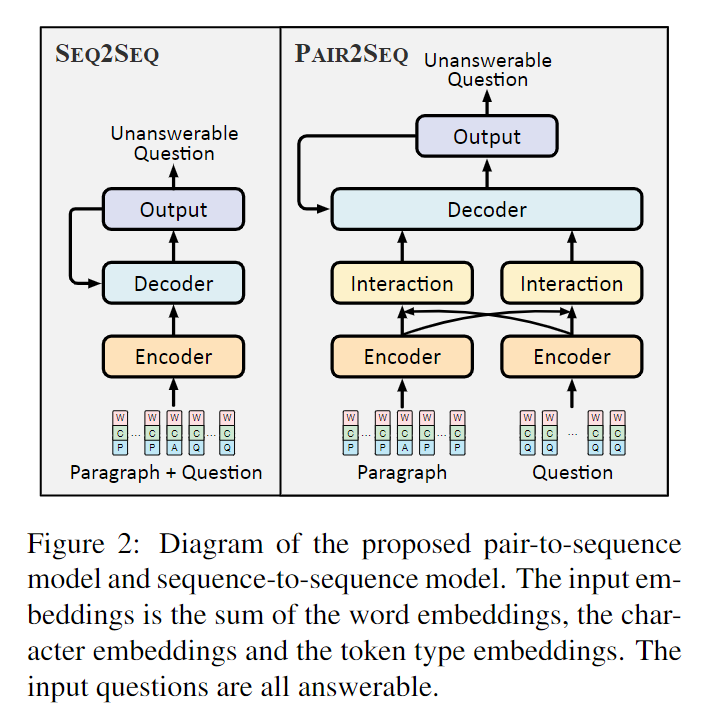### seq2seq

seq2seq模型的输入序列$x$的embedding层由word embeddings、character embeddings和token type embeddings三部分组成，三部分的和为embedding层的输出，且第$i$个token对应的embedding记为$e_i$ 其中token type是为了区分段落与问题，有answer(A)、paragraph(P)和question(Q)三种类型；character embeddings是通过对word embeddings进行最大池化操作所得。

encoder层为单层双向的LSTM，其隐状态$h_i=f_{BiLSTM}\left( h_{i-1},e_i \right)$decoder层为单层单向的LSTM，其隐状态$s_t=f_{LSTM}\left( s_{t-1},\left[ y_{t-1};c_{t-1} \right] \right)$。其中$y_{t-1}$表示$t-1$时刻对应的解码输出，$c_{t-1}$表示$t-1$时刻解码器对应的语义编码，该语义编码是基于attention机制对编码器中的隐状态求和得到的。关于语义编码的相关计算如下所示：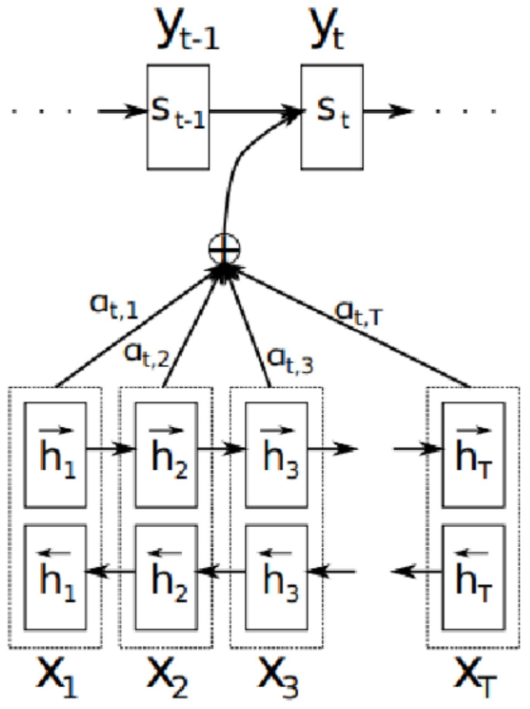### pair2seq

pair2seq模型的embedding层将段落和问题分别编码为$e_{i}^{p}$$e_{j}^{q}$encoder层是两个共享权重的单层双向LSTM，基于embedding层的输入可分别得到段的隐状态$h_{i}^{p}=f_{BiLSTM}\left( h_{i-1}^{p},e_{i}^{p} \right)$问题的隐状态$h_{j}^{q}=f_{BiLSTM}\left( h_{j-1}^{q},e_{j}^{q} \right)$interaction层使用和seq2seq模型中同样的attention机制分别生成意识到问题的段落表示$\tilde{h}_{i}^{p}$意识到段落的问题表示$\tilde{h}_{j}^{q}$

decoder层为单层单向LSTM，其隐状态为

## 实验结果